Скачать презентацию Chapter 8 Relationships Between Inflation Interest Rates and

381f1c96fff3c64dd32eabc2b37b6d87.ppt

• Количество слайдов: 28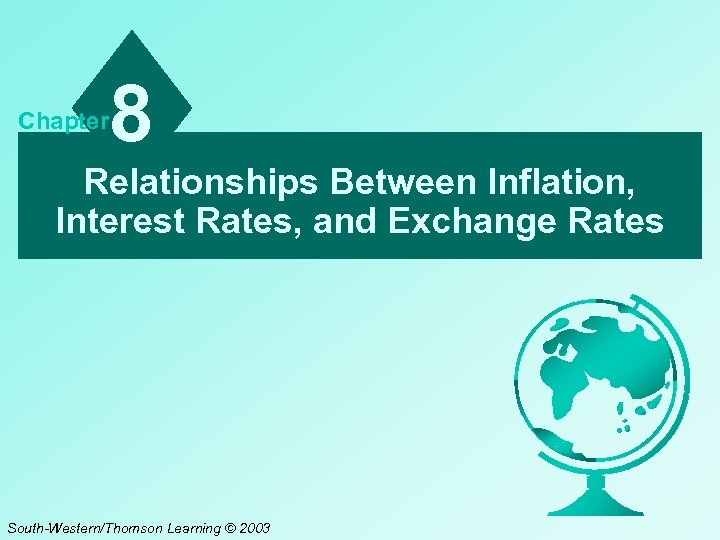Chapter 8 Relationships Between Inflation, Interest Rates, and Exchange Rates South-Western/Thomson Learning © 2003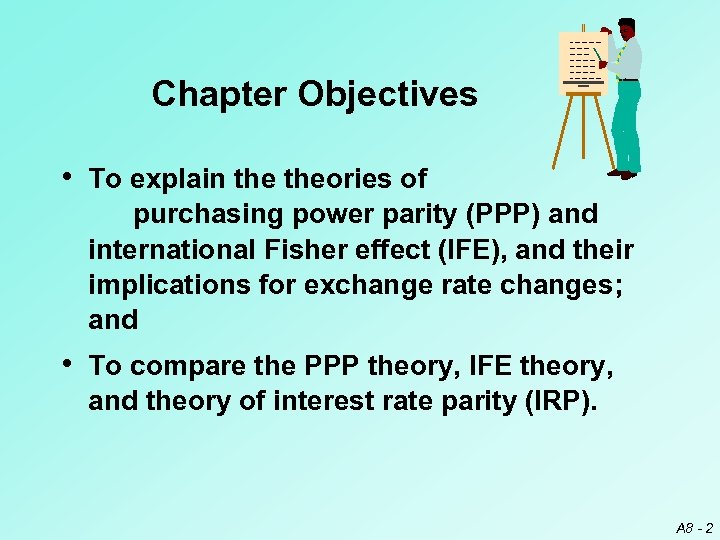Chapter Objectives • To explain theories of purchasing power parity (PPP) and international Fisher effect (IFE), and their implications for exchange rate changes; and • To compare the PPP theory, IFE theory, and theory of interest rate parity (IRP). A 8 - 2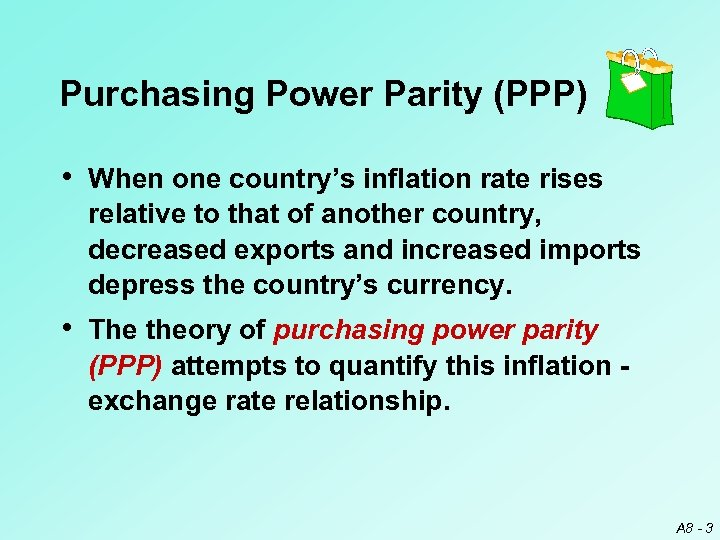Purchasing Power Parity (PPP) • When one country’s inflation rate rises relative to that of another country, decreased exports and increased imports depress the country’s currency. • The theory of purchasing power parity (PPP) attempts to quantify this inflation exchange rate relationship. A 8 - 3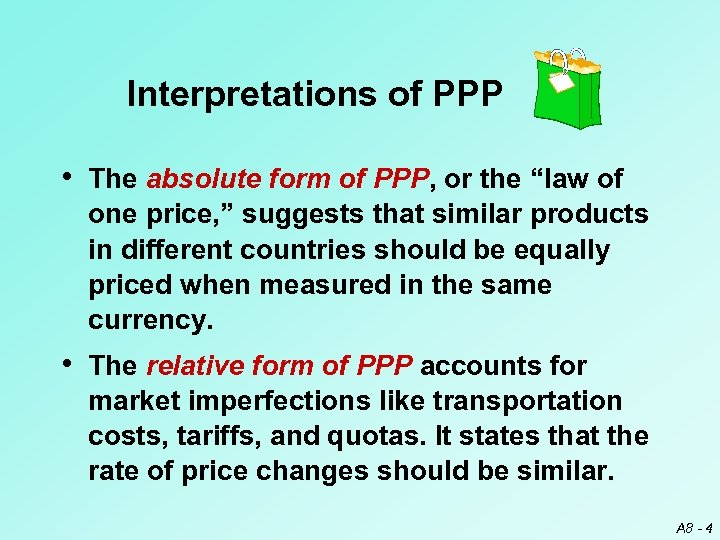Interpretations of PPP • The absolute form of PPP, or the “law of one price, ” suggests that similar products in different countries should be equally priced when measured in the same currency. • The relative form of PPP accounts for market imperfections like transportation costs, tariffs, and quotas. It states that the rate of price changes should be similar. A 8 - 4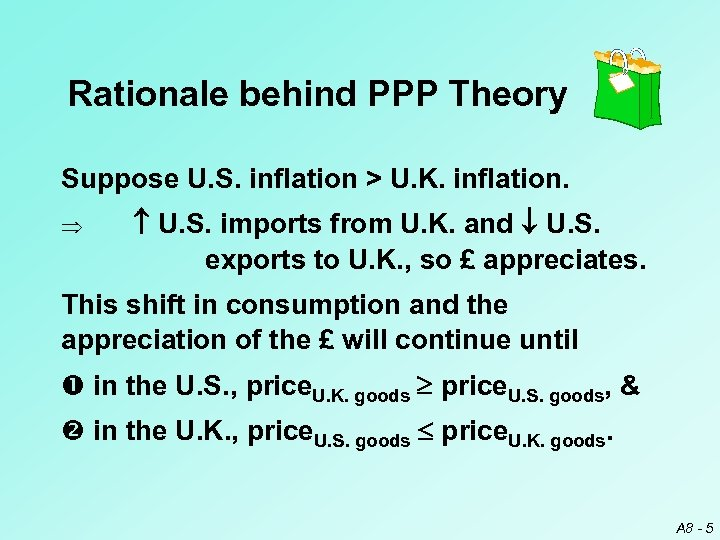Rationale behind PPP Theory Suppose U. S. inflation > U. K. inflation. Þ U. S. imports from U. K. and U. S. exports to U. K. , so £ appreciates. This shift in consumption and the appreciation of the £ will continue until in the U. S. , price. U. K. goods price. U. S. goods, & in the U. K. , price. U. S. goods price. U. K. goods. A 8 - 5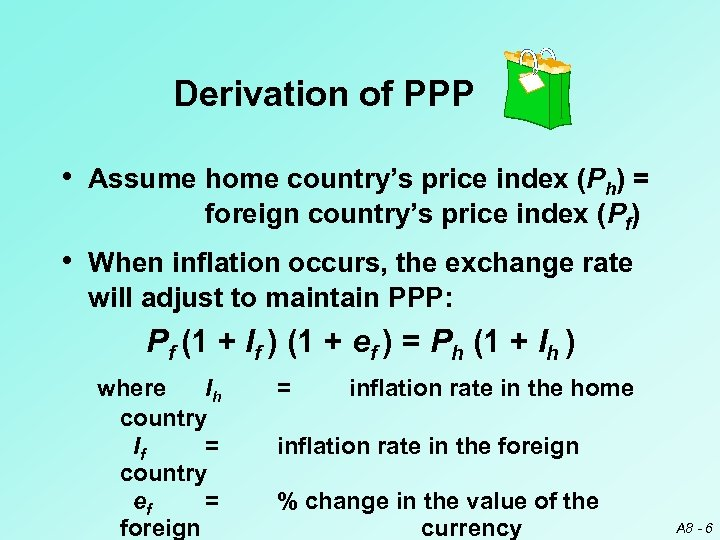Derivation of PPP • Assume home country’s price index (Ph) = foreign country’s price index (Pf) • When inflation occurs, the exchange rate will adjust to maintain PPP: Pf (1 + If ) (1 + ef ) = Ph (1 + Ih ) where Ih country If = country ef = foreign = inflation rate in the home inflation rate in the foreign % change in the value of the currency A 8 - 6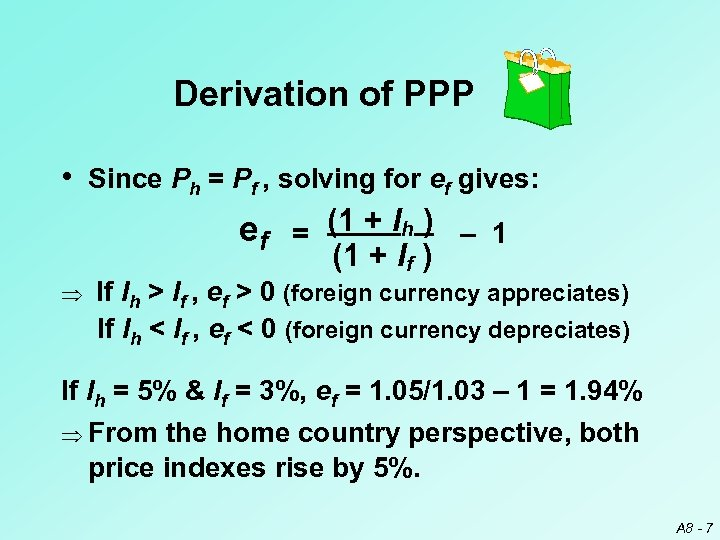Derivation of PPP • Since Ph = Pf , solving for ef gives: ef = (1 + Ih ) – 1 (1 + If ) Þ If Ih > If , ef > 0 (foreign currency appreciates) If Ih < If , ef < 0 (foreign currency depreciates) If Ih = 5% & If = 3%, ef = 1. 05/1. 03 – 1 = 1. 94% Þ From the home country perspective, both price indexes rise by 5%. A 8 - 7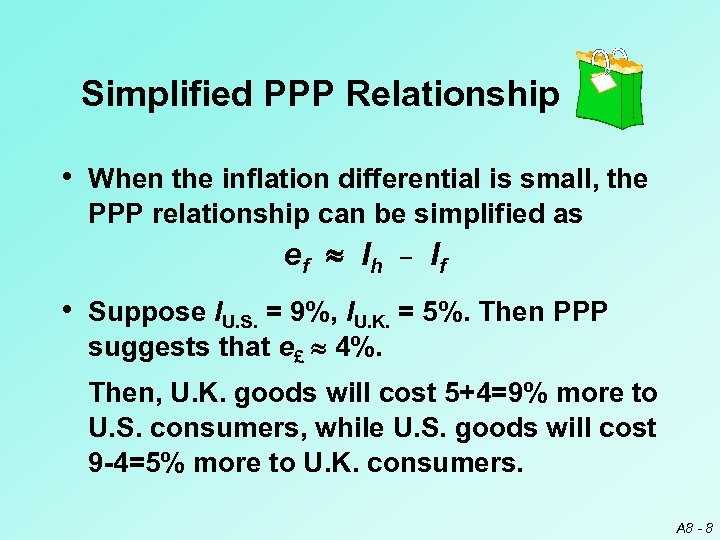Simplified PPP Relationship • When the inflation differential is small, the PPP relationship can be simplified as e f Ih _ If • Suppose IU. S. = 9%, IU. K. = 5%. Then PPP suggests that e£ 4%. Then, U. K. goods will cost 5+4=9% more to U. S. consumers, while U. S. goods will cost 9 -4=5% more to U. K. consumers. A 8 - 8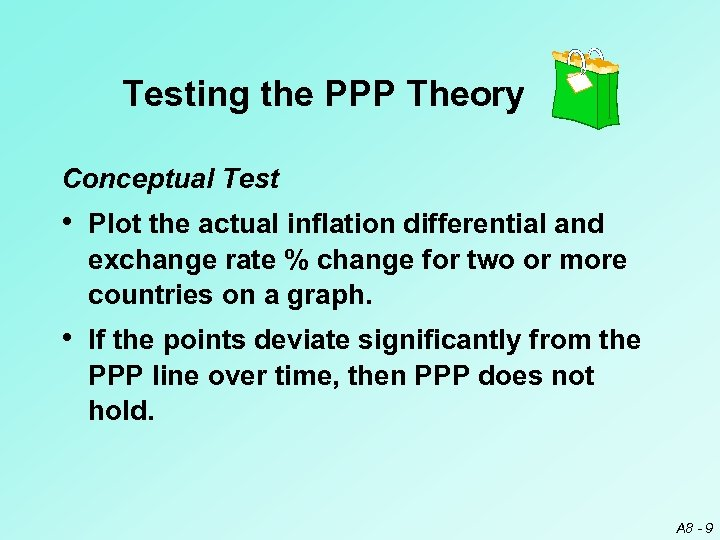Testing the PPP Theory Conceptual Test • Plot the actual inflation differential and exchange rate % change for two or more countries on a graph. • If the points deviate significantly from the PPP line over time, then PPP does not hold. A 8 - 9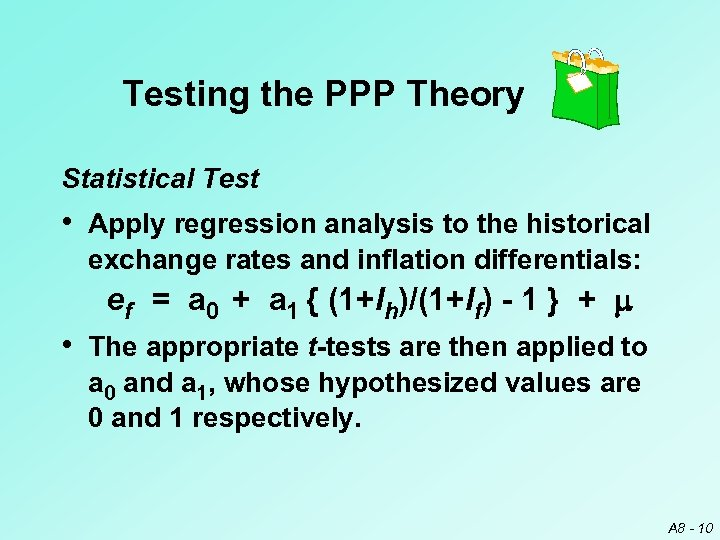Testing the PPP Theory Statistical Test • Apply regression analysis to the historical exchange rates and inflation differentials: ef = a 0 + a 1 { (1+Ih)/(1+If) - 1 } + m • The appropriate t-tests are then applied to a 0 and a 1, whose hypothesized values are 0 and 1 respectively. A 8 - 10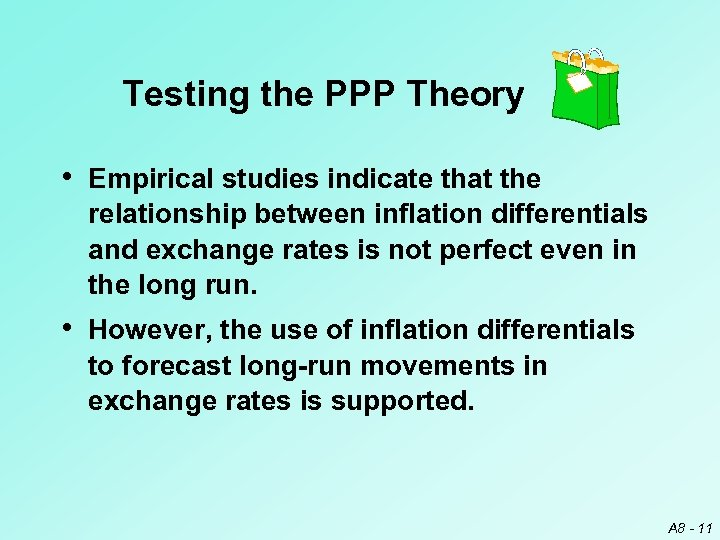Testing the PPP Theory • Empirical studies indicate that the relationship between inflation differentials and exchange rates is not perfect even in the long run. • However, the use of inflation differentials to forecast long-run movements in exchange rates is supported. A 8 - 11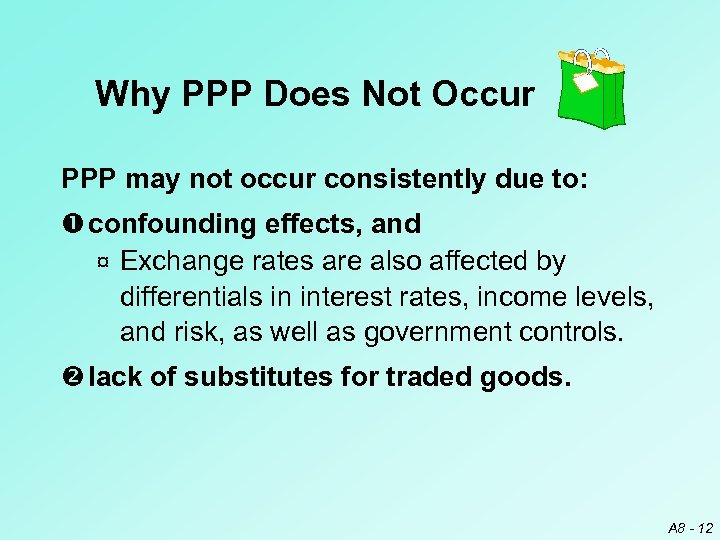Why PPP Does Not Occur PPP may not occur consistently due to: confounding effects, and ¤ Exchange rates are also affected by differentials in interest rates, income levels, and risk, as well as government controls. lack of substitutes for traded goods. A 8 - 12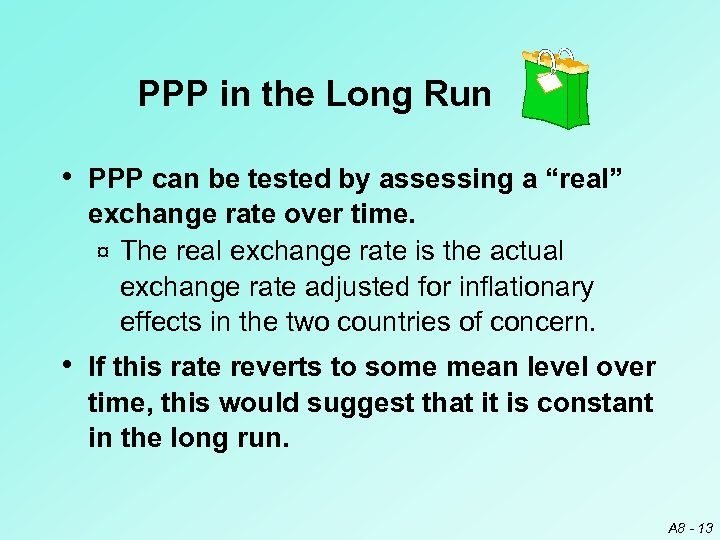PPP in the Long Run • PPP can be tested by assessing a “real” exchange rate over time. ¤ The real exchange rate is the actual exchange rate adjusted for inflationary effects in the two countries of concern. • If this rate reverts to some mean level over time, this would suggest that it is constant in the long run. A 8 - 13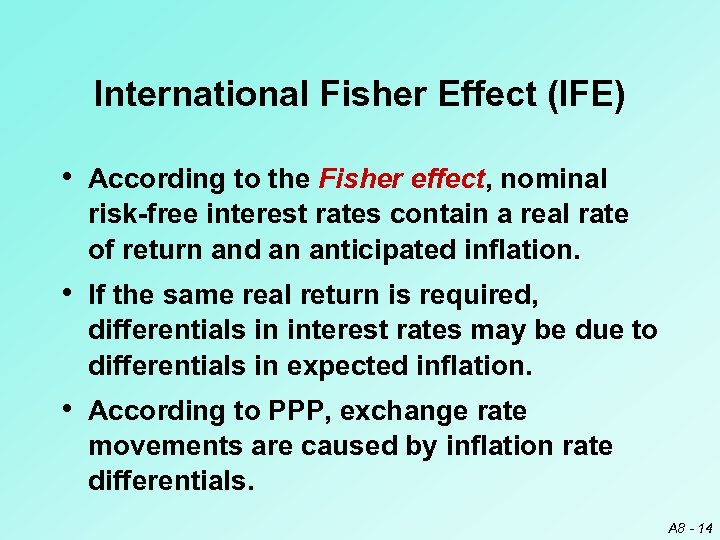International Fisher Effect (IFE) • According to the Fisher effect, nominal risk-free interest rates contain a real rate of return and an anticipated inflation. • If the same real return is required, differentials in interest rates may be due to differentials in expected inflation. • According to PPP, exchange rate movements are caused by inflation rate differentials. A 8 - 14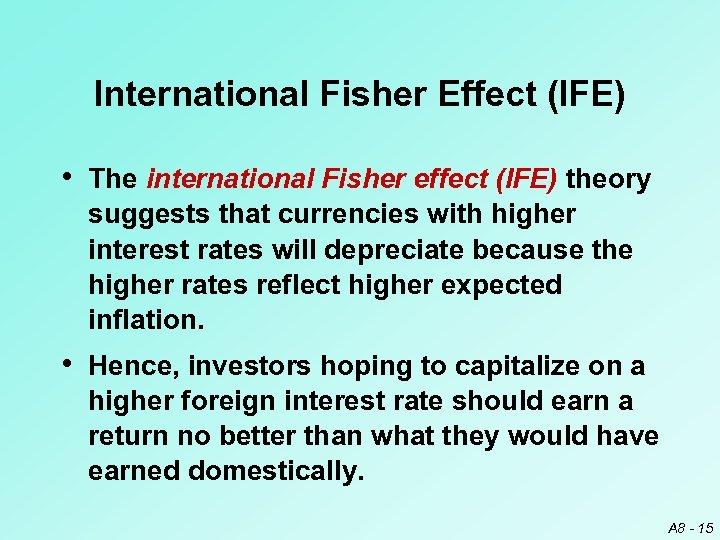International Fisher Effect (IFE) • The international Fisher effect (IFE) theory suggests that currencies with higher interest rates will depreciate because the higher rates reflect higher expected inflation. • Hence, investors hoping to capitalize on a higher foreign interest rate should earn a return no better than what they would have earned domestically. A 8 - 15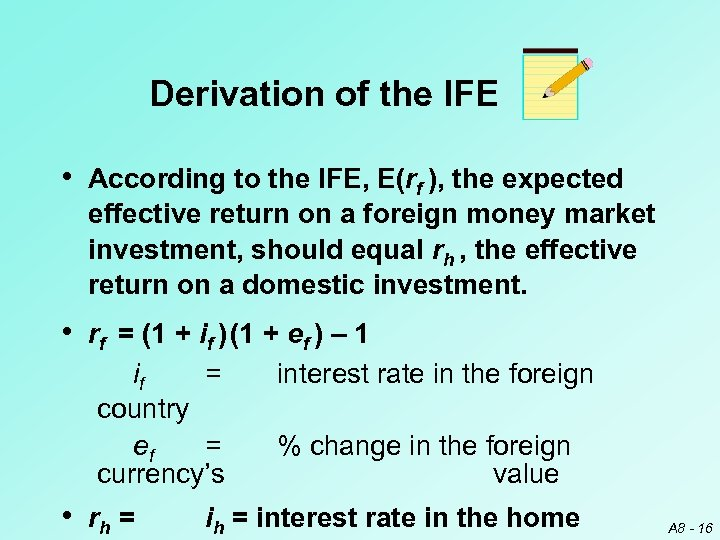Derivation of the IFE • According to the IFE, E(rf ), the expected effective return on a foreign money market investment, should equal rh , the effective return on a domestic investment. • rf = (1 + if ) (1 + ef ) – 1 if = country ef = currency’s • rh = interest rate in the foreign % change in the foreign value ih = interest rate in the home A 8 - 16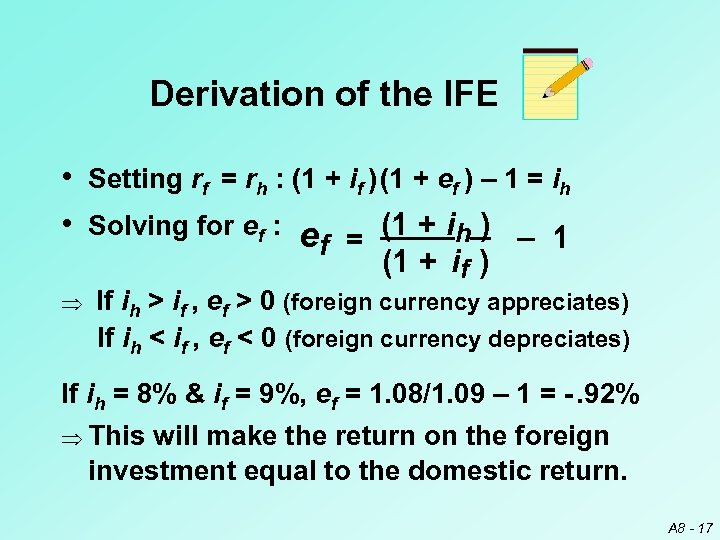Derivation of the IFE • Setting rf = rh : (1 + if ) (1 + ef ) – 1 = ih • Solving for ef : e = (1 + ih ) _ 1 f Þ (1 + if ) If ih > if , ef > 0 (foreign currency appreciates) If ih < if , ef < 0 (foreign currency depreciates) If ih = 8% & if = 9%, ef = 1. 08/1. 09 – 1 = -. 92% Þ This will make the return on the foreign investment equal to the domestic return. A 8 - 17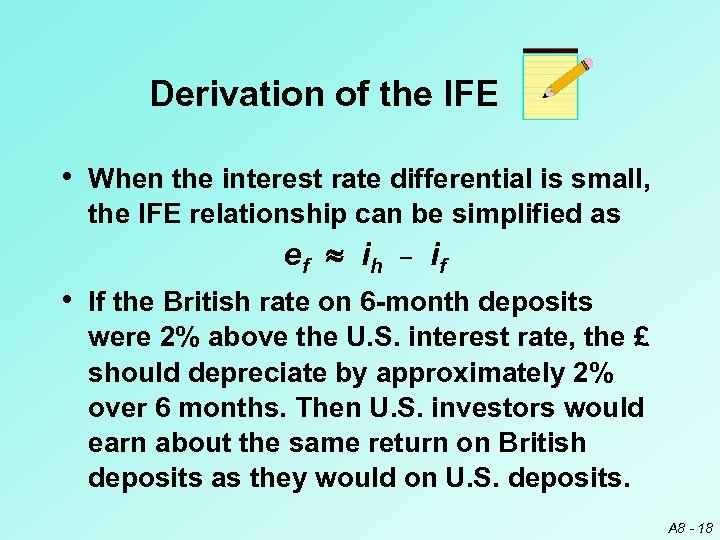Derivation of the IFE • When the interest rate differential is small, the IFE relationship can be simplified as e f ih _ if • If the British rate on 6 -month deposits were 2% above the U. S. interest rate, the £ should depreciate by approximately 2% over 6 months. Then U. S. investors would earn about the same return on British deposits as they would on U. S. deposits. A 8 - 18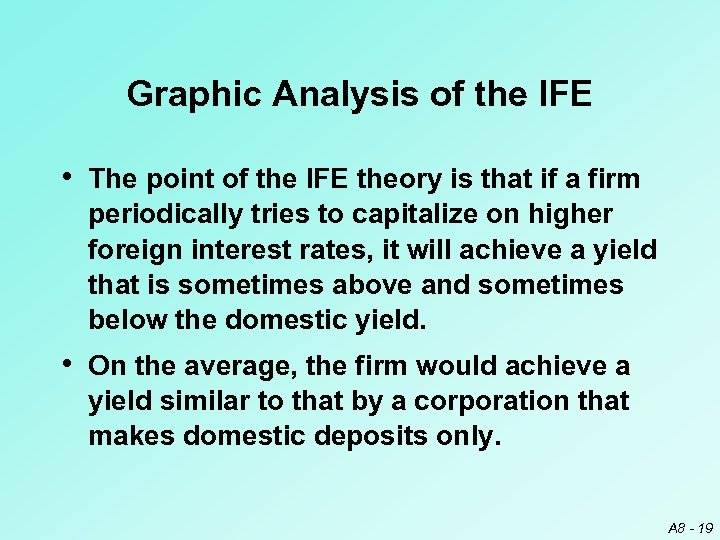Graphic Analysis of the IFE • The point of the IFE theory is that if a firm periodically tries to capitalize on higher foreign interest rates, it will achieve a yield that is sometimes above and sometimes below the domestic yield. • On the average, the firm would achieve a yield similar to that by a corporation that makes domestic deposits only. A 8 - 19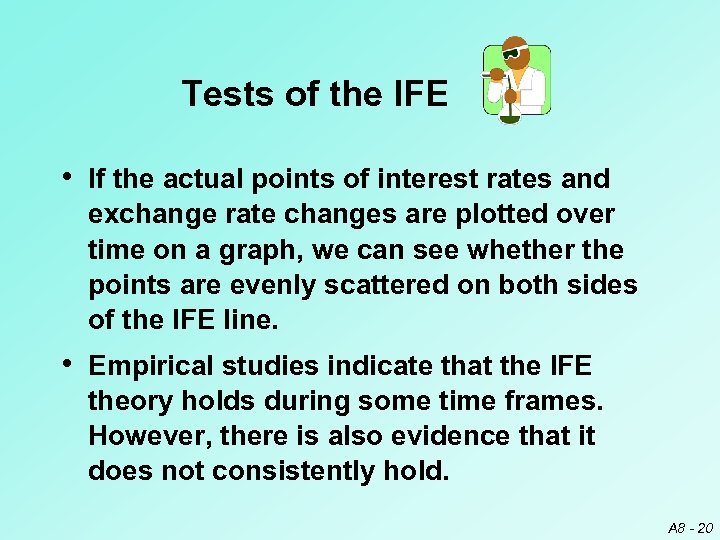Tests of the IFE • If the actual points of interest rates and exchange rate changes are plotted over time on a graph, we can see whether the points are evenly scattered on both sides of the IFE line. • Empirical studies indicate that the IFE theory holds during some time frames. However, there is also evidence that it does not consistently hold. A 8 - 20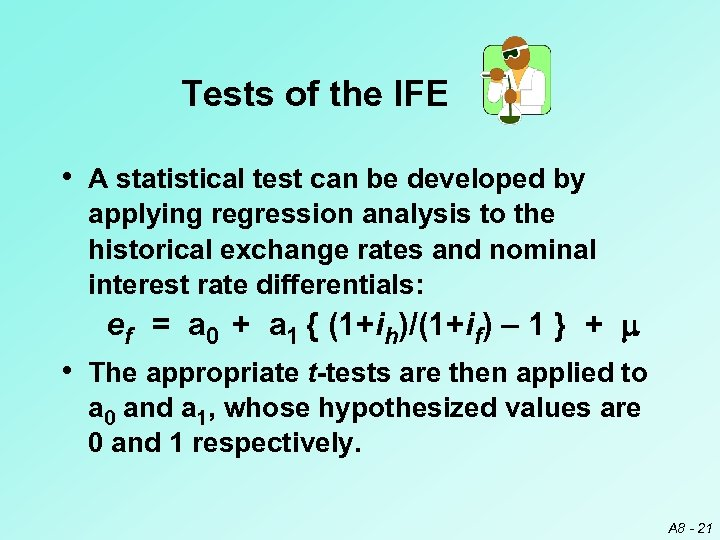Tests of the IFE • A statistical test can be developed by applying regression analysis to the historical exchange rates and nominal interest rate differentials: ef = a 0 + a 1 { (1+ih)/(1+if) – 1 } + m • The appropriate t-tests are then applied to a 0 and a 1, whose hypothesized values are 0 and 1 respectively. A 8 - 21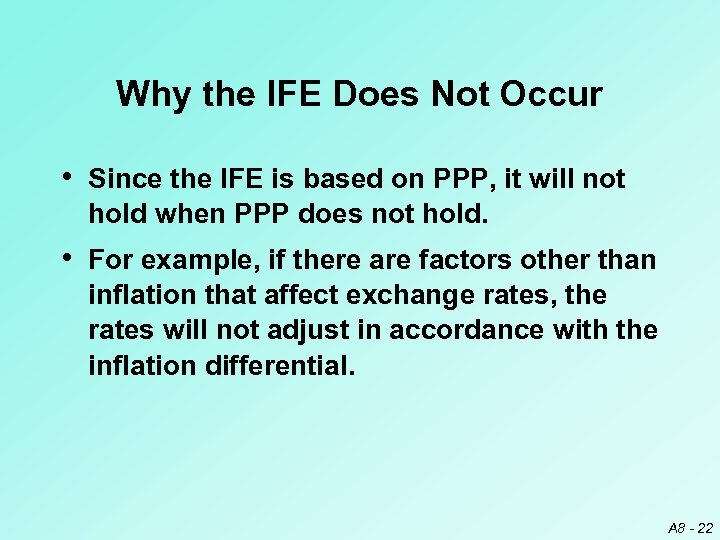Why the IFE Does Not Occur • Since the IFE is based on PPP, it will not hold when PPP does not hold. • For example, if there are factors other than inflation that affect exchange rates, the rates will not adjust in accordance with the inflation differential. A 8 - 22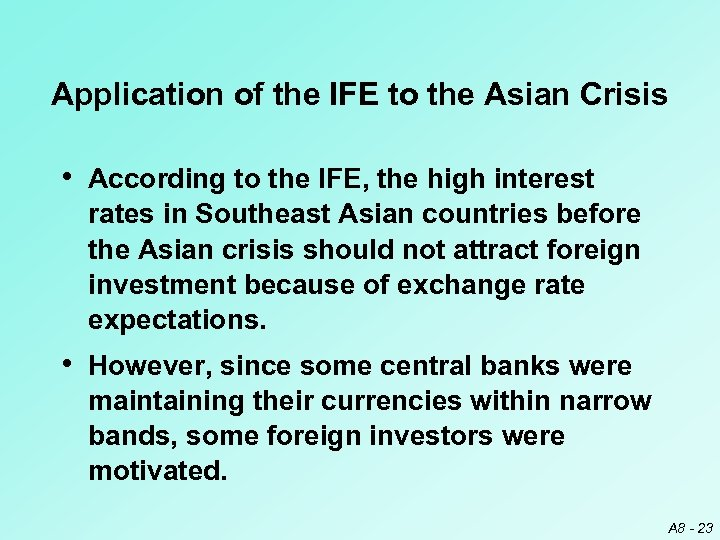Application of the IFE to the Asian Crisis • According to the IFE, the high interest rates in Southeast Asian countries before the Asian crisis should not attract foreign investment because of exchange rate expectations. • However, since some central banks were maintaining their currencies within narrow bands, some foreign investors were motivated. A 8 - 23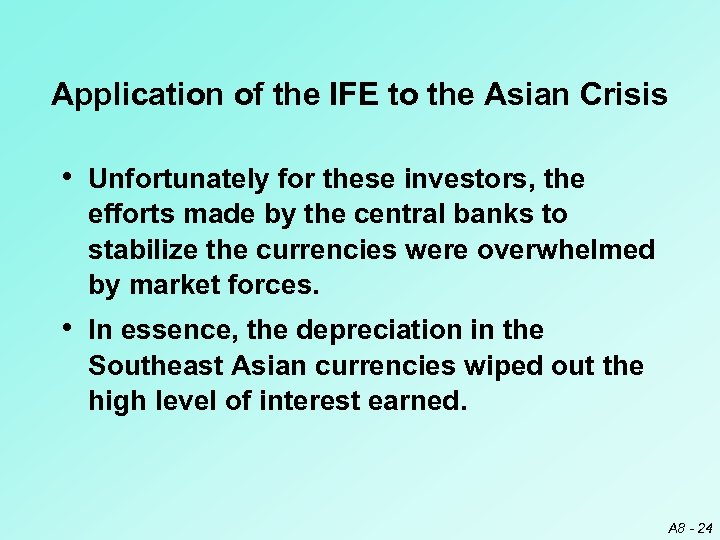Application of the IFE to the Asian Crisis • Unfortunately for these investors, the efforts made by the central banks to stabilize the currencies were overwhelmed by market forces. • In essence, the depreciation in the Southeast Asian currencies wiped out the high level of interest earned. A 8 - 24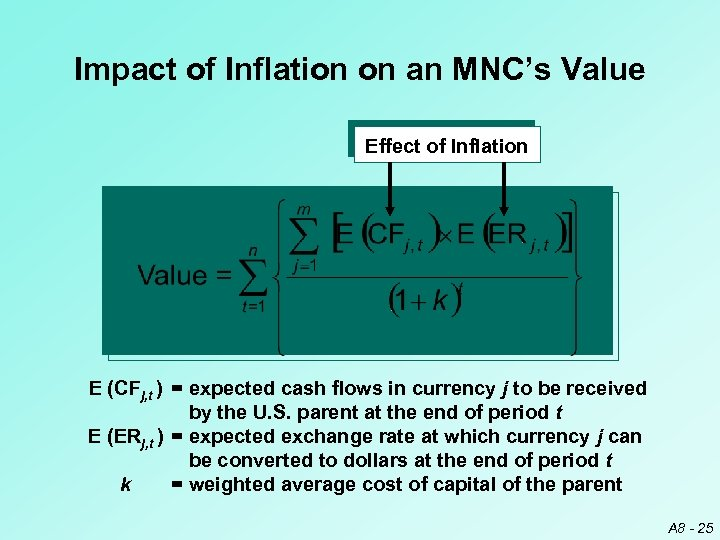Impact of Inflation on an MNC’s Value Effect of Inflation E (CFj, t ) = expected cash flows in currency j to be received by the U. S. parent at the end of period t E (ERj, t ) = expected exchange rate at which currency j can be converted to dollars at the end of period t k = weighted average cost of capital of the parent A 8 - 25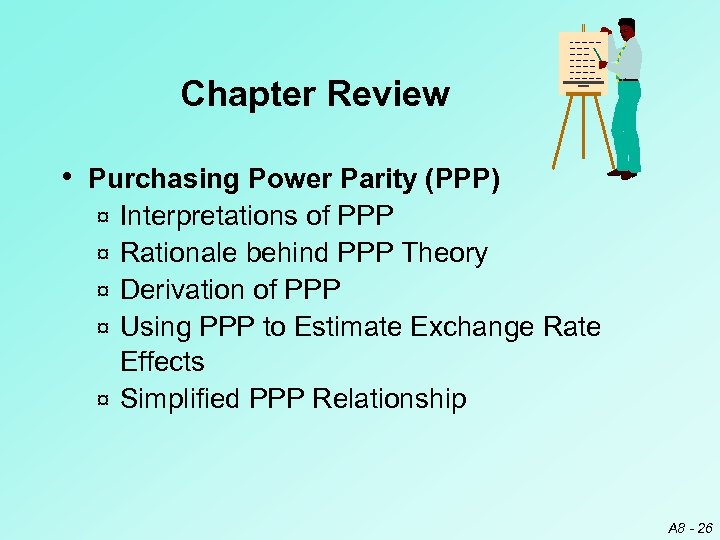Chapter Review • Purchasing Power Parity (PPP) ¤ ¤ ¤ Interpretations of PPP Rationale behind PPP Theory Derivation of PPP Using PPP to Estimate Exchange Rate Effects Simplified PPP Relationship A 8 - 26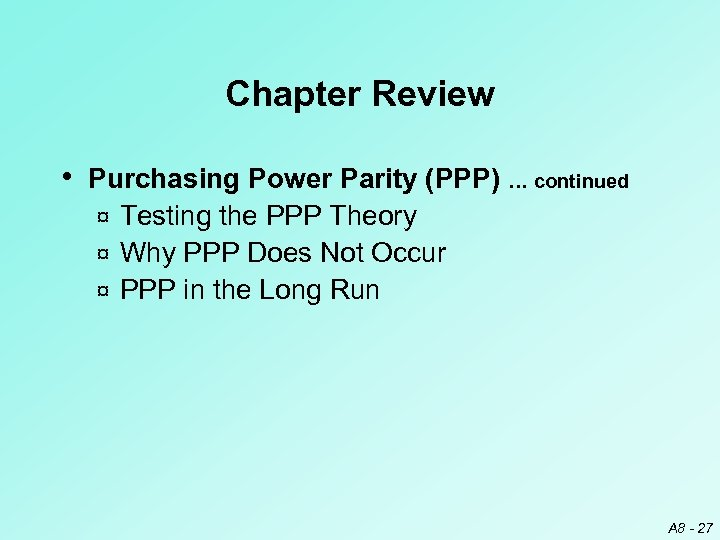Chapter Review • Purchasing Power Parity (PPP) … continued Testing the PPP Theory ¤ Why PPP Does Not Occur ¤ PPP in the Long Run ¤ A 8 - 27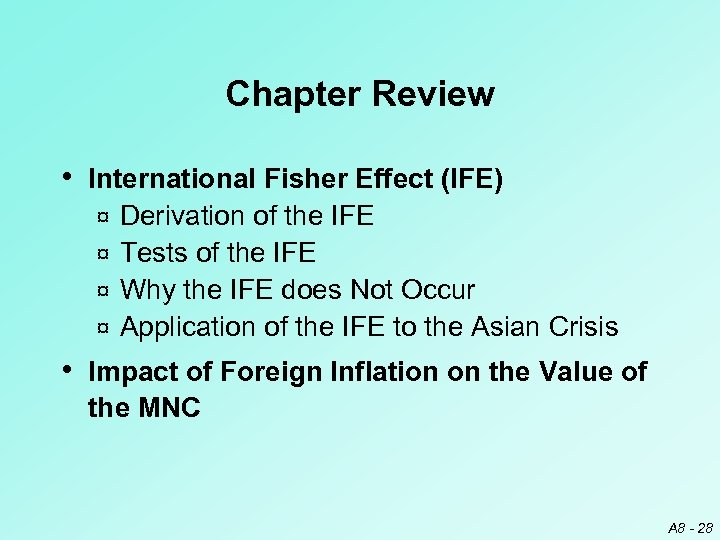Chapter Review • International Fisher Effect (IFE) Derivation of the IFE ¤ Tests of the IFE ¤ Why the IFE does Not Occur ¤ Application of the IFE to the Asian Crisis ¤ • Impact of Foreign Inflation on the Value of the MNC A 8 - 28